# Units + area - math problems

#### Number of problems found: 927

• Wooden prism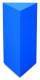Find the weight of a wooden regular triangular prism with a height equal to the perimeter of the base and a figure inscribed in a circle with a radius of 6, M cm, where M is the month of your birth. The density of oak is 680 kg/m3.
• Rectangular lotWhat is the area of a rectangular lot measures 12 3/4 meter long and 8 5/6 wide?
• Find the 29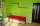Find the area of a room 9/7 m and 2/7 m.
• The length 8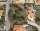The length of a rectangular field is x + 4 and its width is x + 2. what is the area of the field? Answer in polynomials expressions.
• A farmer 4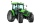A farmer needs to plow a 10 acre field. She start plowing 1/8. how many ares does the framer plow?
• Countertop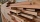Sam it is constructing he measures a rectangle area are on the countertop of 18 1/2 x 35 1/3” he will need to cut his area out in order to place his sink and the opening. What is the product of the two measurements round to the nearest whole?
• Height of the prism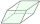The volume of the quadrilateral prism is 723.6 cm3. The base of this prism is a rhombus with a side 9 cm long and a corresponding height of 6.7 cm long. Find the height of the prism.
• A rectangle 8A rectangle measuring 6 cm and 4 cm is enlarged by the ratio 3:1. What is the area of the enlarged rectangle?
• Rectangular field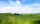What is the area of a rectangular field 100m by 8m?
• Area of a triangle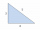Find the area of a triangle with a base of 7 mm and a height of 10 mm?
• The widthThe width of a rectangular garden is 4 m less than the length. If the area of a rectangular garden is 96 square meters, what is the dimension of the garden?
• Semicircle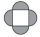The ornament consists of one square and four dark semicircles. The area of the square is 4 cm ^ 2. Find the area of one dark semicircle and round the result to hundreds.
• Touch circle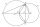Point A has a distance IA, kl = 10 cm from a circle k with radius r = 4 cm and center S. Calculate: a) the distance of point A from the point of contact T if the tangent to the circle is drawn from point A b) the distance of the contact point T from the l
• Markus painterMarkus used ¾ liter of paint to cover 10 ½ square meters of wall. How many liters of paint is needed to cover 12 ¼ square meters of wall?
• A land 2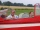A land owner owns two parcels of land measuring 4 2/5 hectares and 6 1/5 hectares. He sells 3/4 of the total land. How many hectares of the land are left?
• AlexandraAlexandra made a rectangular quilt the measured 3 1/4. 2 3/4 feet in width. What is the area of the quilt in square feet? Write an equation to solve.
• A rectangle 7A rectangle that has been split into 24 parts, set in 4 rows of 6 pieces each. The parts in the top 3 rows have been shaded blue. The parts in the left 5 columns have been shaded purple. The first 5 parts in the top 3 rows are striped to show they are sha
• ParallogramThe Parallelogram base is 24 cm and high 10 cm. How many are such tiles required to cover a floor of area 1080m2?
• Matilda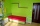Matilda measures the perimeter of her bedroom to determine how many feet of border she needs to go around the tops of the walls. Two walls are each 96 inches long, and two walls are each 3 yards long. She pays \$30 for border that costs \$1 per foot. What a
• A trapezoid 3A trapezoid ABCD has the bases length of a = 120 mm, c = 86 mm and the area A = 2,575 mm2. Find the height of the trapezoid.

Do you have an exciting math question or word problem that you can't solve? Ask a question or post a math problem, and we can try to solve it.

We will send a solution to your e-mail address. Solved examples are also published here. Please enter the e-mail correctly and check whether you don't have a full mailbox.

Units - math problems. Area - math problems.